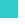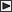BRI Research Paper

No.2

Analysis of Space Frames with Rigid Joints by Generalized Slope Deflection Equation Method.

M.Makino; August, 1960. 21p.

Abstract

The application of matrix calculation in the field of structural analysis are mainly used for the solution of linear equations. However, a row or a column matrix may be equivalent to a vector in a certain vector space. In this case the column matrices of order three are used as the vector quantities in the three dimensional problems.

The first part of this paper shows the derivation of the basic equations in the form of matrix elements under the following conditions:

 a) The members in the frame are assumed straight between joints and the direction of the principal axes of bending and the axis of torsion center on a member in the frame are constant and that these axes meet at a common point. However it may be allowed to vary the width or the depth of the member. b) All displacements are considered small compare to the over-all dimension of the structure so that it is admissible to satisfy the equilibrium conditions in the undeformed rather than in the deformed configuration. c) The length of a member is assumed to be constant under loading. d) The effect of shearing forces due bending is usually ignored, but it may be introduced by a certain modification.

Then the method of analysis is illustrated by means of examples. The first example is taken from a paper by Michalos (1)*, in which the solutions are obtained by moment distribution procedure.

* Number in brackets refer to the reference at the end of the paper.LinksSitemapInquiryJapanese HP

BUILDING RESEARCH INSTITUTE, JAPAN